# System Pumping Requirements

Use this form to calculate the system capacity (flow rate) required to meet the demands of a field.

Net Application Required Per Week:
Total Area:
Total Hours Available to Operate per Day:
hrs
Total Days Available to Operate per Week:
days per week
Irrigation Efficiency:
%
Total Flow Required to Operate System:

## The Equation

This calculator uses this formula to determine the system pumping requirements of a field.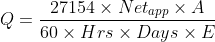Where: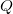= Total flow rate required to operate irrigation system (gpm)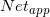= The net application required per week (in)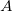= Total area to be irrigated (acres)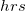= Total hours available to operate per day (hrs)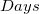= Total days available to operate each week (days)= Efficiency of the irrigation system (as a deciaml)

WSU Prosser - IAREC, 24106 N Bunn Rd, Prosser WA 99350-8694, 509-786-2226,